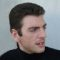Home » Core Java » util » Arrays » Compare two dimensional arraysByron is a master software engineer working in the IT and Telecom domains. He is an applications developer in a wide variety of applications/services. He is currently acting as the team leader and technical architect for a proprietary service creation and integration platform for both the IT and Telecom industries in addition to a in-house big data real-time analytics solution. He is always fascinated by SOA, middleware services and mobile development. Byron is co-founder and Executive Editor at Java Code Geeks.

# Compare two dimensional arrays

With this example we are going to demonstrate how to compare two dimensional arrays. We are using `int `arrays, but the same API applies to any types of arrays, e.g. `boolean[][]`, `byte[][]`, `char[][]`, `double[][]`, `float[][]`,` long[][]`, `short[][]` and `String[][]`. In short, to compare two dimensional arrays we have implemented a method as described below:

• The example’s method is `boolean equal(final int[][] arr1, final int[][] arr2)`. The method takes as parameters two `int` arrays, and returns a `boolean`, that is true if the arrays are equal and false otherwise.
• The method first checks if both the arrays are null, and returns true if they are both null and false otherwise.
• Then the method checks if the two arrays’ lengths are equal. If they are it returns true, or else false.
• Finally, the method invokes the `equals(int[] a, int[] a2)` method of Arrays, for the arrays in the two dimensional arrays. The method returns true if the two specified arrays of ints are equal to one another. Two arrays are considered equal if they contain the same elements in the same order. Also, two array references are considered equal if both are null.
• Create two int arrays, with two dimensions and invoke the example’s method in order to check if they are equal.

Let’s take a look at the code snippet that follows:

```package com.javacodegeeks.snippets.core;

import java.util.Arrays;

public class EqualArrays {

public static void main(String[] args) {

/*
*  Please note that the same API applies to any type of arrays e.g.
*  boolean[][], byte[][], char[][], double[][], float[][], long[][], short[][], String[][] etc
*/

int[][] a1 = {{1, 2, 3}, {5, 19, 56}, {289, 100, 30}};

int[][] a2 = {{1, 2, 3}, {5, 19, 56}, {289, 100, 30}};

if (equal(a1, a2)) {

System.out.println("The arrays are equal!");

} else {

System.out.println("The arrays are not equal");

}

}

public static boolean equal(final int[][] arr1, final int[][] arr2) {

if (arr1 == null) {

return (arr2 == null);

}

if (arr2 == null) {

return false;

}

if (arr1.length != arr2.length) {

return false;

}

for (int i = 0; i < arr1.length; i++) {

if (!Arrays.equals(arr1[i], arr2[i])) {

return false;

}

}

return true;
}
}

```

Output:

``````
The arrays are equal!
```
```

This was an example of how to compare two dimensional arrays in Java.

# Do you want to know how to develop your skillset to become a Java Rockstar?

## Subscribe to our newsletter to start Rocking right now!

### and many more ....0 Followers

Most reacted commentRecent comment authors